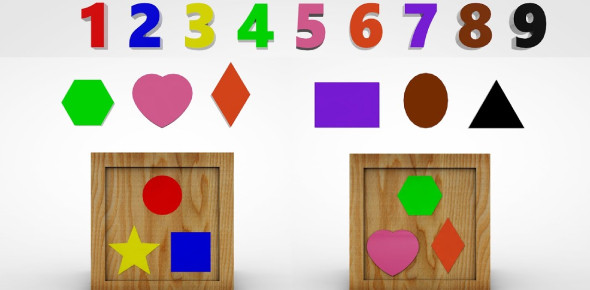# A Basic Maths Quiz About Shapes And Numbers!

17 Questions | Attempts: 282SettingsIn Mathematics, Geometric shapes are the figures which demonstrate the shape of the objects we see in our everyday life. In geometry, shapes are the forms of objects which have boundary lines, angles and surfaces. Take this simple maths quiz about shapes and numbers to know more about the topic. ALL THE BEST!

• 1.
A cube has __________surfaces
• A.

5

• B.

6

• C.

7

• D.

0

• 2.
A cuboid has ______________ edge
• A.

10

• B.

11

• C.

12

• D.

9

• 3.
A cylinder has ______________ vertex
• A.

0

• B.

1

• C.

2

• D.

3

• 4.
A sphere has ___________ curved surface
• A.

0

• B.

1

• C.

2

• D.

3

• 5.
A  cone has ______________ edge
• A.

0

• B.

1

• C.

2

• D.

3

• 6.
A cuboid has ______ plan surface
• A.

5

• B.

6

• C.

7

• D.

8

• 7.
The smallest one-digit number is
• A.

0

• B.

1

• C.

9

• D.

10

• 8.
The largest one-digit number is
• A.

0

• B.

1

• C.

9

• D.

10

• 9.
Add 1 to the greatest 3 digit number
• A.

1

• B.

10

• C.

100

• D.

1000

• 10.
The predecessor of 3459 is
• A.

3450

• B.

3457

• C.

3458

• D.

3460

• 11.
The successor of 3485 is
• A.

3486

• B.

3487

• C.

3484

• D.

3488

• 12.
A line having how many endpoint ______________
• A.

1

• B.

2

• C.

0

• D.

3

• 13.
A line sigment has ______________ number of end point
• A.

1

• B.

2

• C.

3

• D.

Infinite

• 14.
Ray has only _________________ end point
• A.

1

• B.

2

• C.

3

• D.

Infinite

• 15.
8888 write the next number
• A.

8887

• B.

8886

• C.

8889

• D.

None

## Related TopicsBack to top
×

Wait!
Here's an interesting quiz for you.